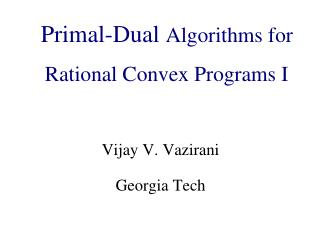DownloadDownload PresentationAlgorithmic Game Theory and Internet Computing

# Algorithmic Game Theory and Internet Computing

Download Presentation## Algorithmic Game Theory and Internet Computing

- - - - - - - - - - - - - - - - - - - - - - - - - - - E N D - - - - - - - - - - - - - - - - - - - - - - - - - - -
##### Presentation Transcript

1. Primal-Dual Algorithms for Rational Convex Programs I Algorithmic Game Theoryand Internet Computing Vijay V. Vazirani Georgia Tech

2. Rational convex program • A nonlinear convex program that always has a rational solution, using polynomially many bits, if all parameters are rational.

3. Rational convex program • A nonlinear convex program that always has a rational solution, using polynomially many bits, if all parameters are rational. • i.e., it “behaves” like an LP!

4. Central Tenet withinMathematical Economics • Markets should operate at equilibrium i.e., prices s.t. Parity between supply and demand

6. Easy if only one good! Domarketsevenadmitequilibriumprices?

7. Supply-demand curves

8. What if there are multiple goods and multiple buyers with diverse desires and different buying power? Domarketsevenadmitequilibriumprices?

9. Irving Fisher, 1891 • Defined a fundamental market model • Special case of Walras’ model

10. amount ofj Concave utility function (for good j) utility

11. total utility

12. For given prices,find optimal bundle of goods

13. Several buyers with different utility functions and moneys.

14. Several buyers with different utility functions and moneys.Equilibrium prices

15. Several buyers with different utility functions and moneys.Find equilibrium prices!

16. Linear utility function utility of i amount ofj

17. Linear Fisher Market • Assume: • Buyer i’s total utility, • mi: money of buyer i. • One unit of each good j. • n buyers and g goods. • Findequilibrium prices!

18. Eisenberg-Gale Program, 1959

19. Eisenberg-Gale Program, 1959 prices pj

20. Why remarkable? • Equilibrium simultaneously optimizes for all agents. • How is this done via a single objective function?

21. Eisenberg-Gale Program Theorem: Optimal soln. gives equilibrium allocations, dual gives prices. Equilibrium utilities and prices are unique. Show: Each buyer spends all her money & buys an optimal bundle of goods. All goods fully sold.

22. KKT conditions

23. Proof of rationality • Guess positive allocation variables (say k). • Substitute 1/pjby a new variable, say qj • LP: with (k + g) unknowns. Constraints: • Inequalities: KKT Conds. (3) & non-negativity. • Equations: KKT Conds. (2) & (4).

24. KKT conditions

25. Combinatorial Algorithm for Linear Case of Fisher’s Model • Devanur, Papadimitriou, Saberi & V., 2002 By extending the primal-dual paradigm to the setting of convex programs & KKT conditions

26. Combinatorial algorithms Yield deep structural insights. Preferable for applications.

27. Auction for Google’s TV ads N. Nisan et. al, 2009: • Used market equilibrium based approach. • Combinatorial algorithms for linear case provided “inspiration”.

28. Yin & Yang

29. An easier question • Given prices p, are they equilibrium prices? • If so, find equilibrium allocations.

30. An easier question • Given prices p, are they equilibrium prices? • If so, find equilibrium allocations. • Equilibrium prices are unique!

31. Bang-per-buck • At prices p, buyer i’s most desirable goods, Si = • Any goods from Siworth m(i) constitute i’s optimal bundle

32. For each buyer, most desirable goods, i.e. m(1) p(1) m(2) p(2) m(3) p(3) m(4) p(4)

33. Network N(p) p(1) m(1) p(2) m(2) t s p(3) m(3) m(4) p(4) infinite capacities

34. Max flow in N(p) p(1) m(1) p(2) m(2) p(3) m(3) m(4) p(4) p: equilibrium prices iff both cuts saturated

35. Idea of algorithm • “primal” variables: allocations • “dual” variables: prices of goods • Approach equilibrium prices from below: • start with very low prices; buyers have surplus money • iteratively keep raising prices and decreasing surplus

36. An important consideration • The price of a good never exceeds its equilibrium price • Invariant: s is a min-cut

37. Invariant: s is a min-cut in N(p) p(1) m(1) p(2) m(2) s p(3) m(3) m(4) p(4) p: low prices

38. AllocationsPrices Idea of algorithm • Iterations: execute primal & dual improvements

40. Fundamental difference betweenLP’s and convex programs • Complementary slackness conditions: involve primal or dual variables, not both. • KKT conditions: involve primal and dual variables simultaneously.

41. KKT conditions

42. KKT conditions

43. Primal-dual algorithms so far(i.e., LP-based) • Raise dual variables greedily. (Lot of effort spent on designing more sophisticated dual processes.)

44. Primal-dual algorithms so far • Raise dual variables greedily. (Lot of effort spent on designing more sophisticated dual processes.) • Only exception: Edmonds, 1965: algorithm for max weight matching.

45. Primal-dual algorithms so far • Raise dual variables greedily. (Lot of effort spent on designing more sophisticated dual processes.) • Only exception: Edmonds, 1965: algorithm for max weight matching. • Otherwise primal objects go tight and loose. Difficult to account for these reversals -- in the running time.

46. Our algorithm • Dual variables (prices) are raised greedily • Yet, primal objects go tight and loose • Because of enhanced KKT conditions